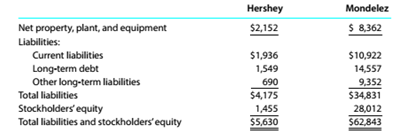Chapter 9, Problem 9.15E

Chapter
Section
Textbook Problem

Debt ratio, ratio of liabilities to stockholders' equity, and ratio of fixed assets to long-term liabilities Recent balance sheet information lor two companies in the snack food industry. The Hershey Company (HSY) and Mondelez International. Inc. (MDLZ). is as follows (in millions of dollars):a. Determine the debt ratio for both companies. Round to one decimal place. b.Determine the ratio of liabilities to stockholders' equity for both companies. Round to one decimal place. c.Determine the ratio of fixed assets to long-term liabilities for both companies. Round to two decimal places. d. Interpret the ratio differences between the two companies.

To determine

(a)

Introduction:

Accounting ratios are used to evaluate the financial performance of the business organisation

Solvency analysis:

It is used to evaluate company's ability to pay its long-term debts. It also helps the owner to determine the chances of firm's long-term survival.

To calculate:

Debt ratio of both the companies.

Explanation
 Particulars HSY MDLZ Total liabilities $4,175$34,831 Total Assets $5,630$62,843 Debt ratio formula Debit ratio= total debttotal assets
To determine

(b)

Solvency analysis:

Solvency analysis is used to evaluate companies' ability to pay its long-term debt, it also helps owner to determine the chances of firm's long-term survival.

To calculate:

The debt to equity ratio of both the companies

To determine

(c)

Solvency analysis:

Solvency analysis is used to evaluate companies' ability to pay its long-term debt, it also helps owner to determine the chances of firm's long-term survival.

The ratio of fixed assets to long term liabilities

To determine

(d)

Introduction:

Solvency analysis:

Solvency analysis is used to evaluate companies' ability to pay its long-term debt, it also helps owner to determine the chances of firm's long-term survival.

To explain:

The ratio differences between both the companies.

Still sussing out bartleby?

Check out a sample textbook solution.

See a sample solution

The Solution to Your Study Problems

Bartleby provides explanations to thousands of textbook problems written by our experts, many with advanced degrees!

Get Started

Find more solutions based on key concepts